# Data Node Glossary

Browse our Data Node Terms.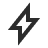# Automation

Sends an email notification to a list of recipients when triggered.

### Create Viz

Creates a new Data App when triggered.

### Source Change Trigger

Sets off action nodes in the Dataflow when a source is modified.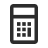# Calculate

### Summarize

Creates a new table of summarized values.

### Field Calculator

Adds new columns of values that are calculated from other columns.

### Average

Calculates the average from a set of input numbers.

### Min

Returns the minimum value of the selected number column in the input.

### Max

Returns the maximum value of the selected number column in the input.

### Sum

Adds the total of all input numbers or values or adds all the values in a column.

### Cosine

A function that provides the length of the adjacent side divided by the hypotenuse length.

### Sine

A function that provides the input number of a ratio between the long side (hypotenuse) and the side that is counter to an acute angle in a right triangle.

### Absolute

Removes the negative sign (-) in front of a number. It displays a positive number.

### Ceiling

Rounds the input number to the highest integer number closest to the input.

### Floor

Rounds the input number to the lowest integer number closest to the input.

### Round

Replaces the input number with the nearest integer number.

### Divide

Divides two single numbers (of any type) or two columns of numbers.

### Multiply

Multiples two single numbers (of any type) or two columns of numbers.

### Subtract

Subtract two singles (of any type) or two columns of numbers.

Adds two single numbers (of any type) or two columns of numbers.

### Text

An editable text field that can be changed.

### Number

A number that can be changed into a decimal (float), integer, or an unassigned integer.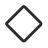# Control Flow

### Less Than

Output is true if the value of inputA is less than the value of inputB.

### Bigger Than

Output is true if the value of inputA is bigger than the value of inputB.

### Is Equal

Output is true if the value of inputA and inputB are the same.

### If

Evaluates if the condition of an input value is true or false.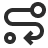# Convert

Sends an email notification to a list of recipients when triggered.

### Create Viz

Creates a new Data App when triggered.

### Source Change Trigger

Sets off action nodes in the Dataflow when a source is modified.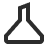# Experiment

### Stable Random

Produces a random number that remains the same regardless if the node is re-evaluated.

### Random Number

Produces a random number that regenerates every time the node is evaluated.‍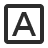# Generate

### Unit

Generates a unit of measurement.

### Date

Generates a Date value.

### Currency

Generates a value of currency.

### Boolean

Generates a true or false value.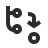# Transform

### Text Transform

Uppercase or lowercase a text column.

### Reorder Columns

Reorders a set of columns in a table.

### Remove Rows

Remove rows from the top or bottom of a table.

### Ungroup

Remove groups from an input table.

### Text Truncate

Takes several characters from the left or right side of the text as a new column.

### Split

Splits a column into multiple columns using a delimiter value.

### Remove Columns

Remove rows from the top or bottom of a table.

### Keep Rows

Keep rows at the top or bottom of a table.

### Join

Combines columns, entire tables, or both by joining them based on the columns and join direction selected.

### Group Count

Calculates the number of groups in a table.

### Find and Replace

Replaces the value of a cell for all rows that match a search condition.

### Edit Column

Change the type, name, or type and name of columns.

### Data Mapper

Divides two single numbers (of any type) or two columns of numbers.

### Combine Columns

Renames a set of columns from a table.

### Column Rename

Renames a set of columns from a table.

### Clean Up

Trim columns and remove blank rows.

### Breakout

Extracts a set of columns from a table.

### Append

Append rows together, sharing columns with the same name and type.

### Revit Data

Picks data from a Revit source.

### JSON To Table

Transforms JSON file to a table.

### Find In JSON

Locates text string within a JSON file.

### Filter JSON

Filters a JSON file.

Pick header row by index and infer types from the following rows. Also, setting zero keeps headers but re-evaluates column types.

### String Concatenate

Combines two text fields together.

### Concatenate

Multiples two single numbers (of any type) or two columns of numbers.

### Pluck Column

Selects and copies a specific column from a table.

### Element Index

Outputs the position (index) of a value in a table.

### Repeat

Displays appended copies of the input value.

### Range

Displays all the rows between two specific positions.

### Unique

Extracts the distinct values from an input array.

### Count

Calculates the number of rows in a table.

### Place As Index

Outputs the row at a specific index in a table.

### Index Of Element

Provides the index of a specific value (opposite to ElementAtIndex).

### Element At Index

Gives the position (index) of a value in a table.

### Sort

Uses selected fields to sort table records in ascending or descending order.

### Filter

Filters inputs based on values in a column.

### Group By

Groups table records based on the selected field.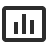# Visualize

### Treemap Chart

Treemap charts visualize data as a hierarchical set of nested rectangles. Each branch of the tree has a rectangle that is tiled with smaller rectangles denoting sub-branches.

### Stacked Bar Chart

Stacked bar chart shows how a larger section is divided into smaller sections and what the relation of each part has on the total amount.

### Scorecard Chart

Use scorecards to graphically represents your data. Scorecards are a great way to highlight a particular piece of information.

### Pie Chart

A pie chart is a circle that is divided into sectors that represent a proportion of the whole.

### Line Chart

Line charts show data plotted as a range of data points called markers. Line charts are helpful to show how specific data changes over equal periods.

### Horizontal Bar Chart

Horizontal bar charts work the same way as traditional bar charts except that they’re oriented horizontally.

### Gauge Chart

Displays the input data as a gauge chart within the Dataflow. Use this tool to inspect your data.

### Funnel Chart

Funnel charts visualize the progressive reduction of data as it goes from one phase to another. Data in each phase is categorized as different portions of the whole (100% of the total).

### Donut Chart

Donut charts function similarly to pie charts, but they can provide a better data intensity ratio since the circle’s center isn’t there.

### Bar Chart

A bar chart contains rectangular bars with lengths proportional to the values that they represent. While one axis of a bar chart compares particular categories, the other axis represents a distinct value.

### Area Chart

Area charts are similar to line charts, except the area below the line is filled in with color. They represent the changes in quantitative values over equal periods.

### Table View

Displays the input data in a tabular format. Use this tool to inspect your data as you build a Dataflow.